# Soft-IntroVAE

Project site for Soft-IntroVAE

Project maintained by taldatech Hosted on GitHub Pages — Theme by mattgraham

# CVPR 2021 Oral

### Technion - Israel Institute of Technology

####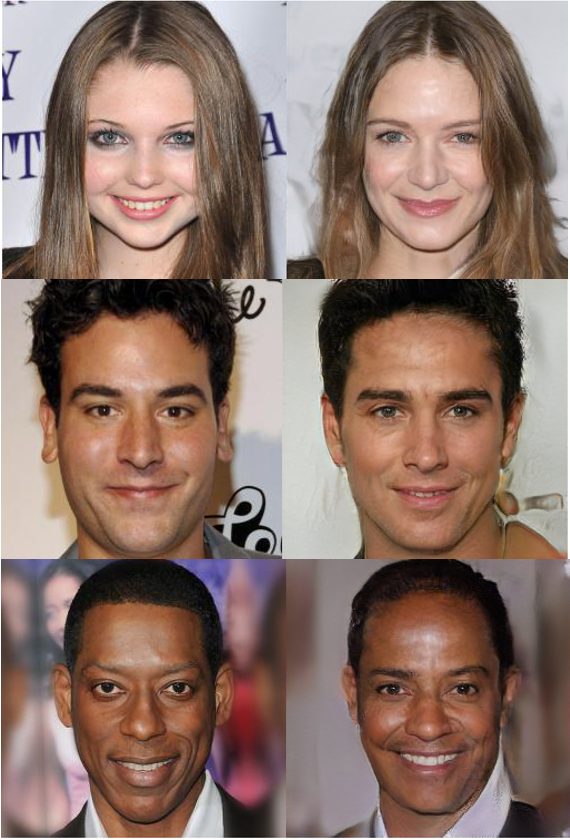# Soft-IntroVAE

Soft-IntroVAE: Analyzing and Improving Introspective Variational Autoencoders
Tal Daniel, Aviv Tamar

Abstract: The recently introduced introspective variational autoencoder (IntroVAE) exhibits outstanding image generations, and allows for amortized inference using an image encoder. The main idea in IntroVAE is to train a VAE adversarially, using the VAE encoder to discriminate between generated and real data samples. However, the original IntroVAE loss function relied on a particular hinge-loss formulation that is very hard to stabilize in practice, and its theoretical convergence analysis ignored important terms in the loss. In this work, we take a step towards better understanding of the IntroVAE model, its practical implementation, and its applications. We propose the Soft-IntroVAE, a modified IntroVAE that replaces the hinge-loss terms with a smooth exponential loss on generated samples. This change significantly improves training stability, and also enables theoretical analysis of the complete algorithm. Interestingly, we show that the IntroVAE converges to a distribution that minimizes a sum of KL distance from the data distribution and an entropy term. We discuss the implications of this result, and demonstrate that it induces competitive image generation and reconstruction. Finally, we describe two applications of Soft-IntroVAE to unsupervised image translation and out-of-distribution detection, and demonstrate compelling results.

### Overview

Variational Autoencoder (VAE) is a generative model that belongs to the explicit density models family. VAEs use Variational Inference (VI) to optimize a lower bound on $$\log p_{\theta}(x)$$, called the evidence lower bound (ELBO) as follows:

$\log p_{\theta}(x) \geq \mathbb{E}_{q_{\phi}}\left[\log p_{\theta}(x \mid z) \right] - D_{KL}[q_{\phi}(z \mid x) \mid \mid p(z)] \triangleq ELBO(x;\phi,\theta),$

where $$q_{\phi}(z \mid x)$$ is termed the encoder and $$p_{\theta}(x \mid z)$$ is termed the decoder.

For the encoder, $$E_{\phi}$$, and decoder, $$D_{\theta}$$, the objective of VAE, which is maximized, is written as follows:

$\mathcal{L}_{E_{\phi}}(x) = ELBO(x),$ $\mathcal{L}_{D_{\theta}}(x) = ELBO(x).$

For an in-depth tutorial on VAEs (theory and code), please visit 046202 - Unsupervised Learning and Data Analysis GitHub repository.

We introduce Soft-IntroVAE, a VAE that is trained adversarially in an introspective manner (i.e., no discriminator is needed).

The objective of Soft-IntroVAE, which is maximized, is written as follows:

$\mathcal{L}_{E_{\phi}}(x,z) = ELBO(x) - \frac{1}{\alpha}\exp \left( \alpha ELBO\left(D_{\theta}(z) \right) \right),$ $\mathcal{L}_{D_{\theta}}(x,z) = ELBO(x) +\gamma ELBO \left( D_{\theta}(z) \right),$

where $$\alpha$$ and $$\gamma$$ are hyperparameters which are set to $$\alpha=2$$ and $$\gamma=1$$ in all our experiments.

This objective portrays a game between the encoder and the decoder: the encoder is induced to distinguish, through the ELBO value, between real and generated samples, while the decoder is induced to generate samples the `fool’ the encoder.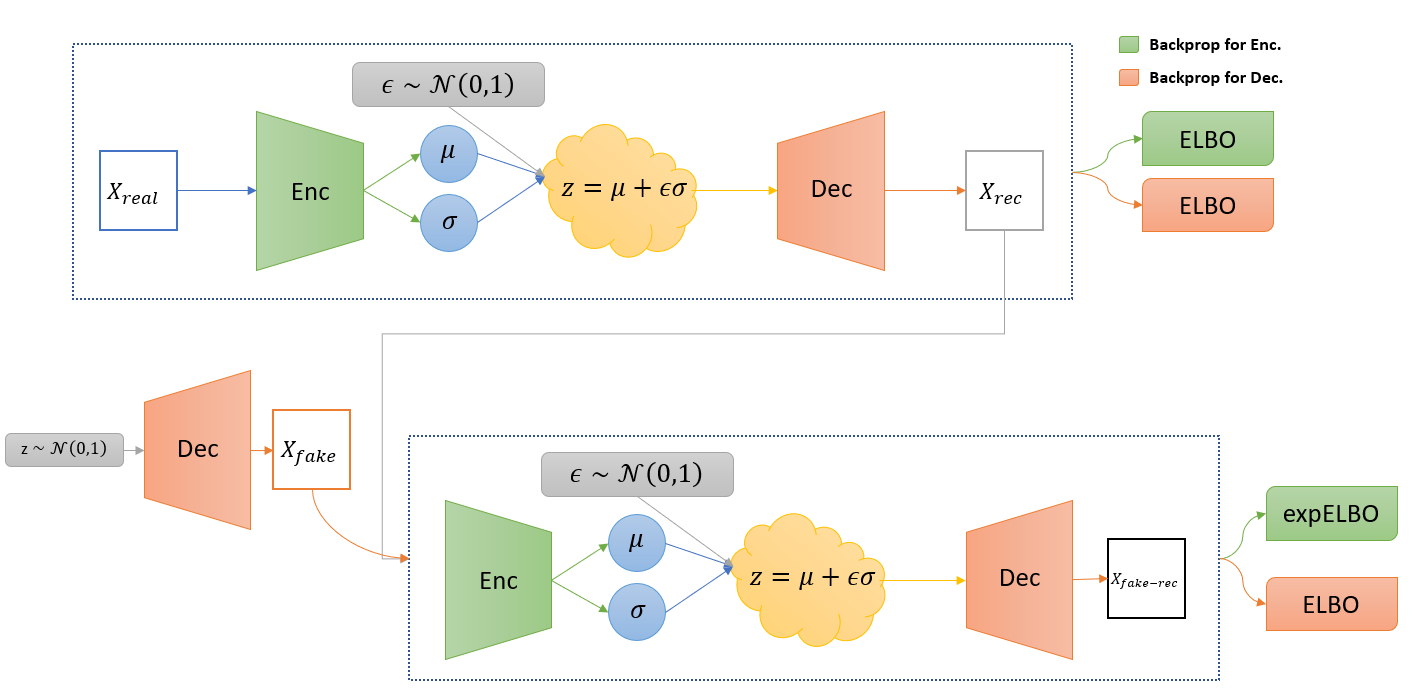### Main Theoretical Result

Our main result, which we analyze in the paper, is that unlike GANs, the S-IntroVAE model does not converge to the data distribution, but to an entropy-regularized version of it. Mathematically, representing the encoder as $$q \doteq q(z|x)$$ and the decoder as $$d \doteq p_d(x|z)$$, the optimal decoder satisfies:

$d^* \in \argmin_d \{KL(p_{data} || p_d) \} + \gamma H(p_d(x)) ,$

where $$H(p_d(x))$$ denotes the Shannon entropy.

In the paper, we prove that for $$q^{*} = p_{d^{*}}(z|x)$$, the tuple $$( q^{*}, d^{*} )$$ is a Nash equilibrium of the game.

### Implementation

We provide code and Jupyter Notebook tutorials at our official GitHub repository.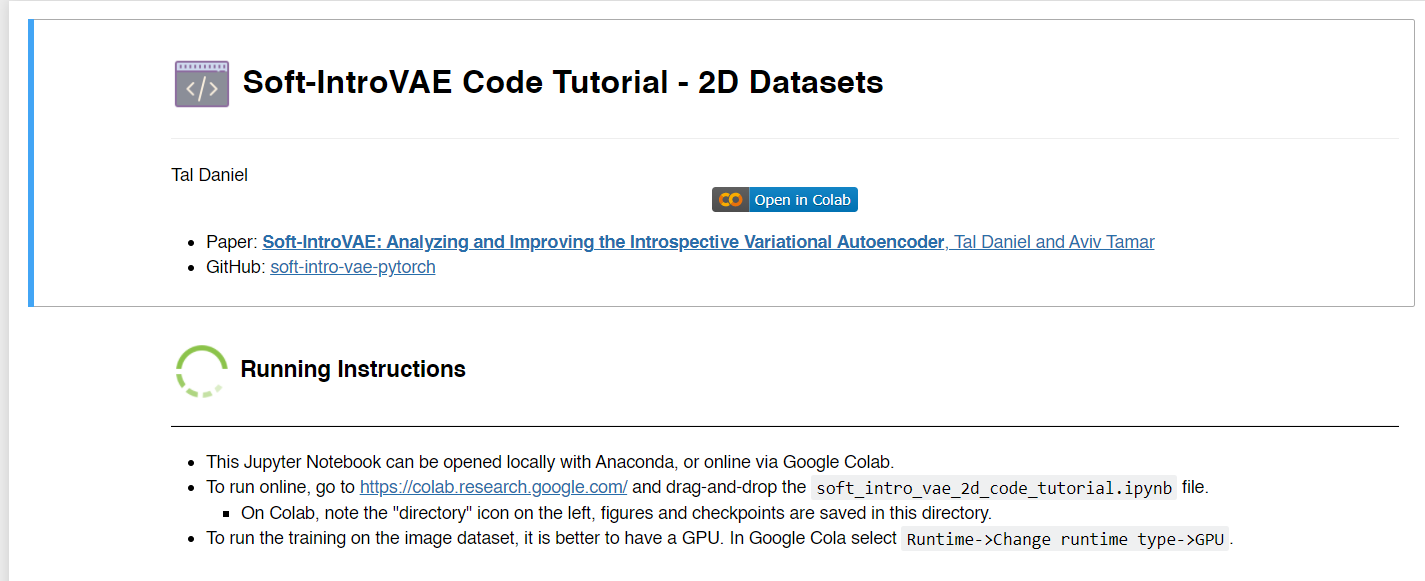### Results

#### 2D Datasets

We evaluate our method on four 2D datasets: 8 Gaussians, Spiral, Checkerboard and Rings, and compare with a standard VAE and IntroVAE. In the following figures we plot random samples from the models and a density estimation, obtained by approximating $$p(x)$$ with $$\exp(ELBO)$$.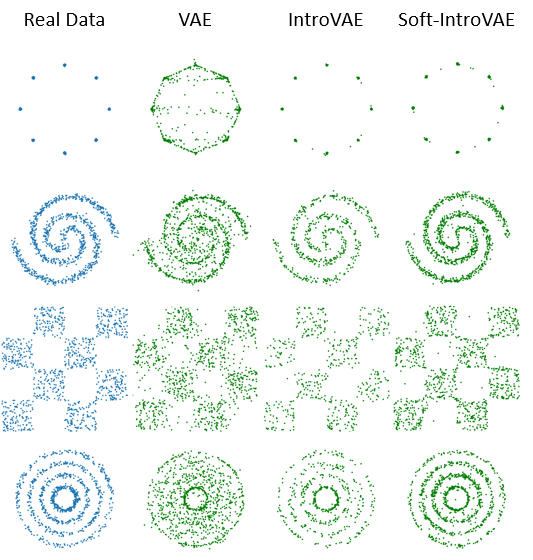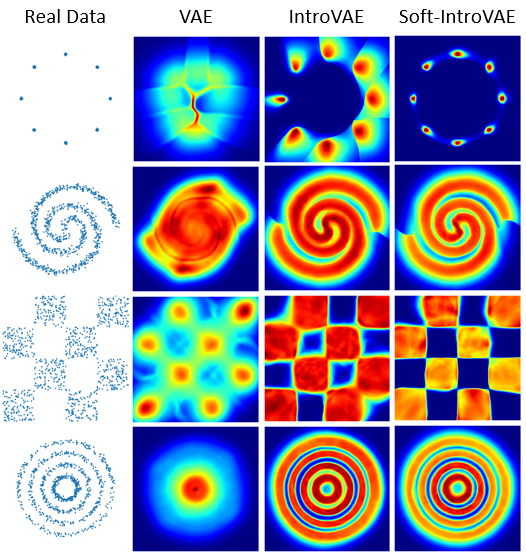#### Image Datasets

We evaluate Soft-IntroVAE on image synthesis in terms of both inference (i.e., reconstruction) and sampling, and experiment with two convolution-based architectures: IntroVAE’s  encoder-decoder architecture with residual-based convolutional layers and ALAE’s  style-based autoencoder architecture, which adopted StyleGAN’s style generator to a style-based encoder. At the head of this page, we show samples from the style-based Soft-IntroVAE when trained on FFHQ and reconstructions of test data when trained on CelebA-HQ, both at 256x256 resolution. In the following figures, we show samples (left) and reconstructions of test data (right) from the residual-based convolutional Soft-IntroVAE when trained on CIFAR10.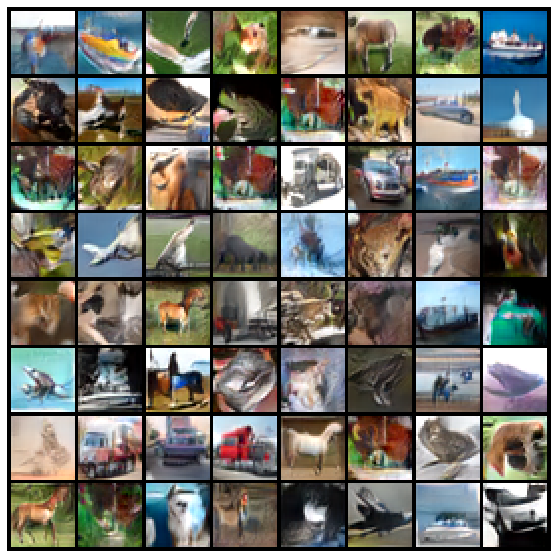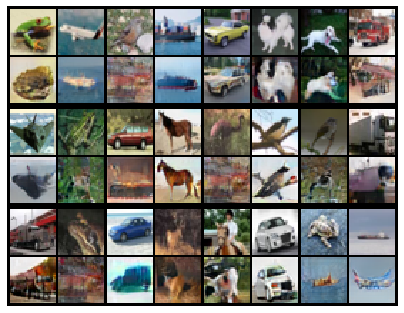Next, the following figure shows smooth interpolation between the latent vectors of two images from S-IntroVAE trained on the CelebA-HQ dataset.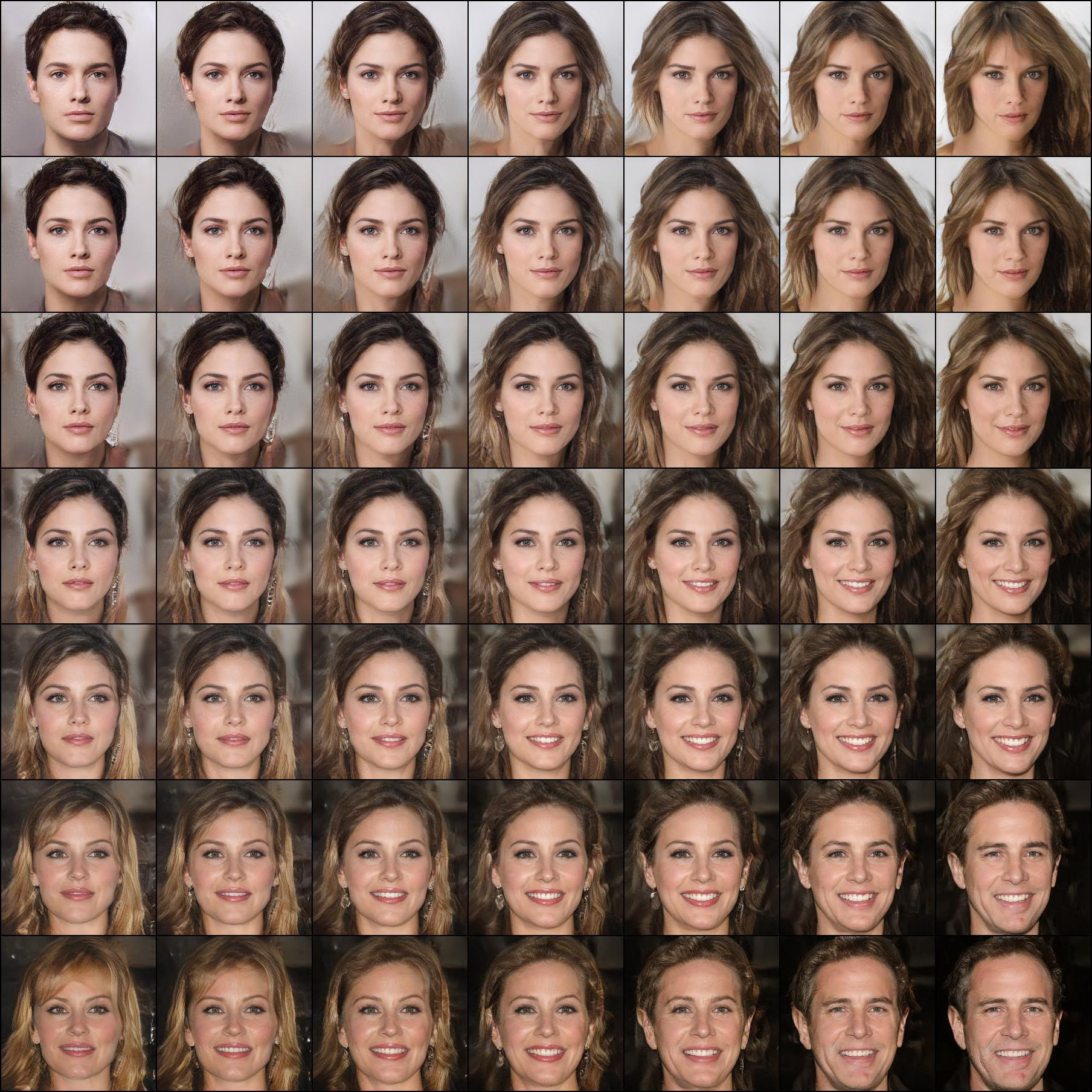### Other Applications

#### Image Translation

We evaluate Soft-IntroVAE on image translation, the task of learning disentangled representations for class and content, and transferring content between classes (e.g. given two images of cars from different visual classes, rotate the first car to be in the angle of the second car, without altering the car visualization). Our focus is unsupervised image translation, where no labels are used at any point. We adopt the two-encoder architecture proposed in LORD , where one encoder is for the class and the other for the content. The separation to two encoders imposes strong inductive bias, as it explicitly learns different representations for the class and content. Content transfer is performed by taking a pair of images $$(x_i, x_j)$$, encoding them to $$([z_i^{class}, z_i^{content}], [[z_j^{class}, z_j^{content}])$$ and then exchanging the content latents such that the input to the decoder is $$([z_i^{class}, z_j^{content}], [[z_j^{class}, z_i^{content}])$$. This is depicted in the following figures: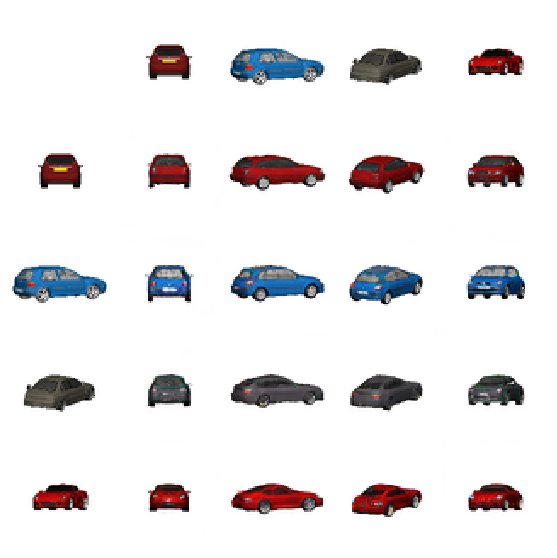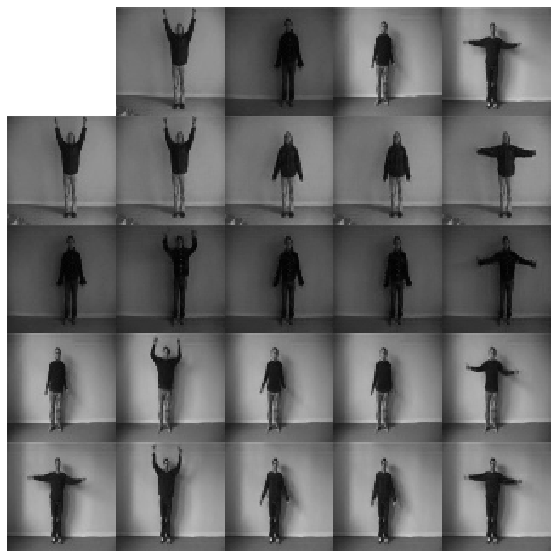#### Out-of-Distribution (OOD) Detection

One common application of likelihood-based generative models is detecting novel data, or out-of-distribution (OOD) detection. Typically in an unsupervised setting, where only in-distribution data is seen during training, the inference modules in these models are expected to assign in-distribution data high likelihood, while OOD data should have low likelihood. Surprisingly, Nalisnick et al.  showed that for some image datasets, density-based models, such as VAEs and flow-based models, cannot distinguish between images from different datasets, when trained only on one of the datasets. We use Soft-IntroVAE to estimate the log-likelihood of the data, using importance-weighted sampling from the trained models. In the following figures, histogram of log-likelihoods is shown when the models are trained on CIFAR10, where the left figure is of the standard VAE and the right is of Soft-IntroVAE. It can be seen that using the standard VAE, samples from SVHN are assigned higher likelihood than the likelihood of the original data (CIFAR10) aligning with the findings of , while Soft-IntroVAE correctly assigns higher likelihoods to the designated data.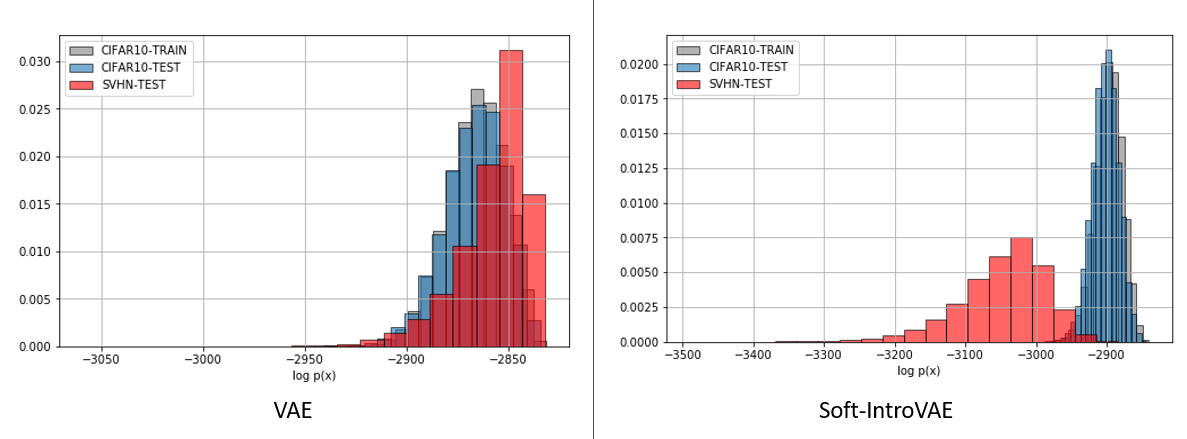#### 3D Point Cloud Generation

To further demonstrate that Soft-IntroVAE is capable of handling different data types and architectures, we combine a PointNet-based  encoder with a fully-connected decoder, to learn 3D point clouds from the ShapeNet dataset. The resulting model can reconstruct test samples and generate new ones. Following is an interpolation in the latent space between an airplane and a car.### Gotta Catch ‘Em All

Well done for reaching thus far! Here is a bonus: we curated a “Digital Monsters” dataset: ~4000 images of Pokemon, Digimon and Nexomon (yes, it’s a thing) and trained S-IntroVAE. In the figures below, on the left is a sample from the (very diverse) dataset (we used augmentations to enrich it), and on the right, samples generated from S-IntroVAE. We hope this does not give you nightmares.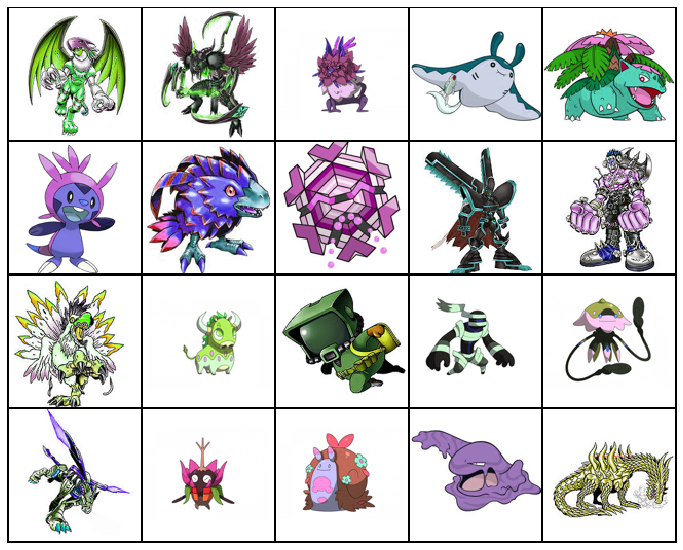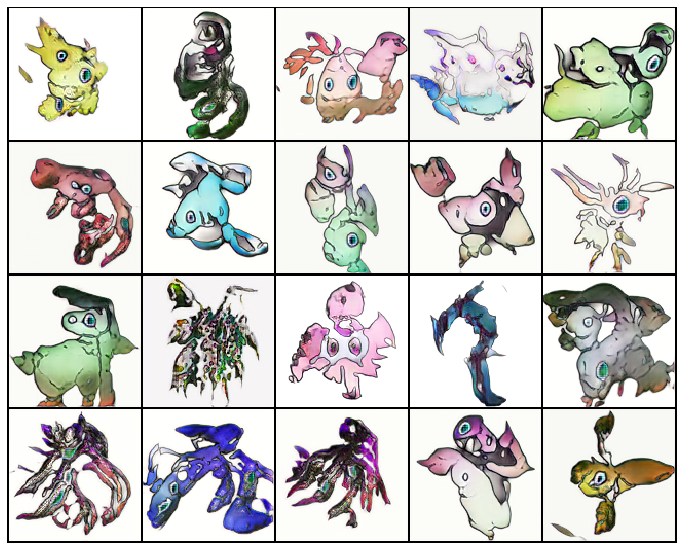1. Huaibo Huang, Zhihang Li, Ran He, Zhenan Sun, and Tie-niu Tan. Introvae: Introspective variational autoencoders forphotographic image synthesis. In Proceedings of the 32nd International Conference on Neural Information Processing Systems, NIPS’18.
2. Aviv Gabbay and Yedid Hoshen. Demystifying inter-class disentanglement. In International Conference on Learning Representations, 2019.
3. Eric Nalisnick, Akihiro Matsukawa, Yee Whye Teh, Di-lan Gorur, and Balaji Lakshminarayanan. Do deep generative models know what they don’t know?. In International Conference on Learning Representations, 2019.
4. Stanislav Pidhorskyi, Donald A. Adjeroh, and Gianfranco Doretto. Adversarial Latent Autoencoders. In Proceedings of the IEEE Computer Society Conference on Computer Vision and Pattern Recognition (CVPR), 2020.
5. Qi, Charles R., et al. Pointnet: Deep learning on point sets for 3d classification and segmentation. Proceedings of the IEEE conference on computer vision and pattern recognition. 2017.